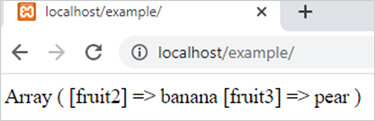array_diff_uassoc() function in php || PHP Functions# array_diff_uassoc() function in php

1486

array_diff_uassoc() function of PHP is used to calculate the difference of two or more associative arrays by using a user define function. It is an inbuilt function of PHP.

Syntax:

array_diff_uassoc (\$arr1, \$arr2, \$arr3, ...,\$arrN, function);

Here

1. \$arr1 is the array which values is compared to others.
2. \$arr2,\$arr3 and \$arrN are the arrays which values is compared to first array and the different values of first array is return as resulting array.
3. function is a user define function. We calculate the result with the help of this function

Example 1:

```<?php
function user_function(\$x,\$y)
{
if (\$x===\$y)
{
return 0;
}
return (\$x>\$y)?1:-1;
}
\$arr1=array("fruit1"=>"mango","fruit2"=>"banana","fruit3"=>"pear");
\$arr2=array("fruit4"=>"orange","fruit1"=>"mango","fruit3"=>"grapes");
\$result=array_diff_uassoc(\$arr1,\$arr2,"user_function");
print_r(\$result);
?>```

Output:Share:## Big Hosting Days

Upto 75@ OFF on Web Hosting

## Get a .COM for just \$6.98

Secure Domain for a Mini Price